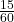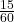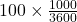The Nardo ring is a circular test track for cars. It has a circumference of 12.5km. Cars travel around the track at a constant speed of 100k

Question

The Nardo ring is a circular test track for cars. It has a circumference of 12.5km. Cars travel around the track at a constant speed of 100km/h. A car starts at the easternmost point of the ring and drives for 15 minutes at this speed.
1. What distance, in km, does the car travel?
2. What is the magnitude of the car’s displacement, in km, from its initial position?
3. What is the speed of the car in m/s?

in progress 0
5 months 2021-09-01T07:16:03+00:00 1 Answers 2 views 0

1. 25 Km

2. zero

3. 27.7 m/s

Explanation:

Data provided in the question:

Circumference of the track = 12.5 km

Speed of the car = 100 Km/h

Time for which car travels = 15 minutes =hr

Now,

1. Distance traveled = Speed × Time

= 100 ×= 25 Km

2. The distance traveled is 2 times the circumference of the track (i.e 2 × 12.5 = 25 Km)

Which means that the car is again at the initial position

Therefore, The displacement is zero.

3. Speed of car in Km/hr = 100 Km/h

now,

1 Km = 1000 m

1 hr = 3600 seconds

therefore,

100 Km/h =m/s

= 27.7 m/s

Hence, the speed of car in m/s = 27.7